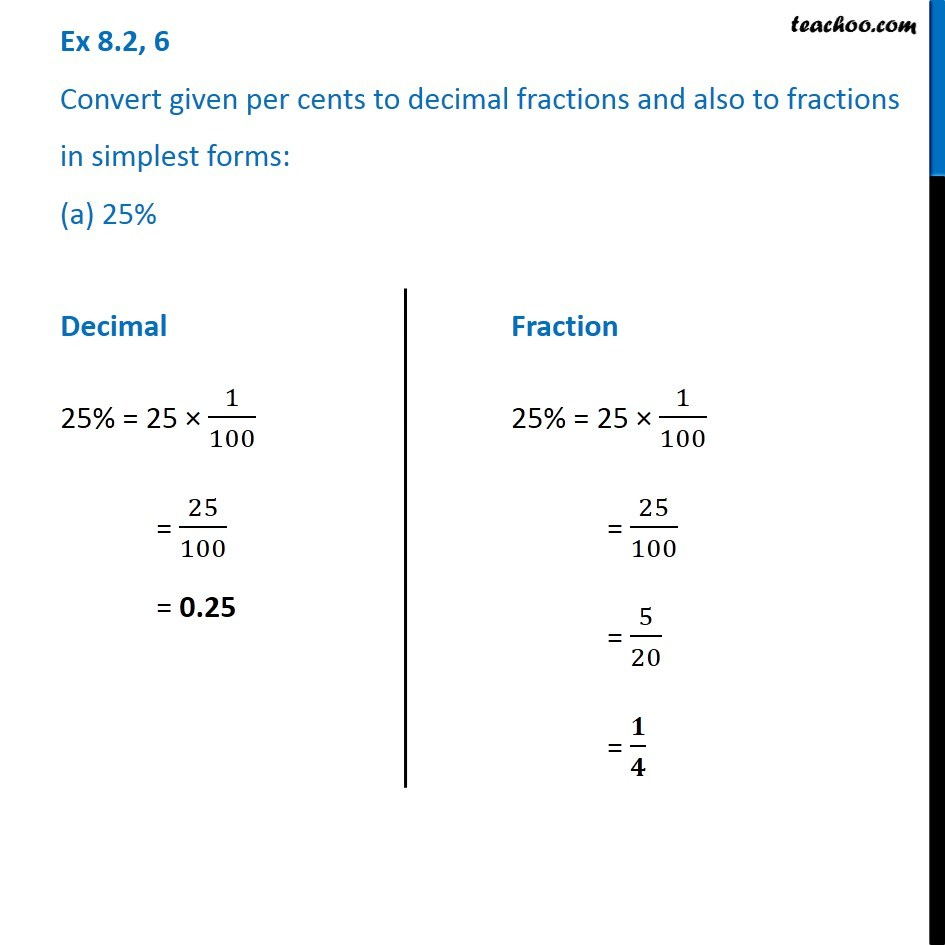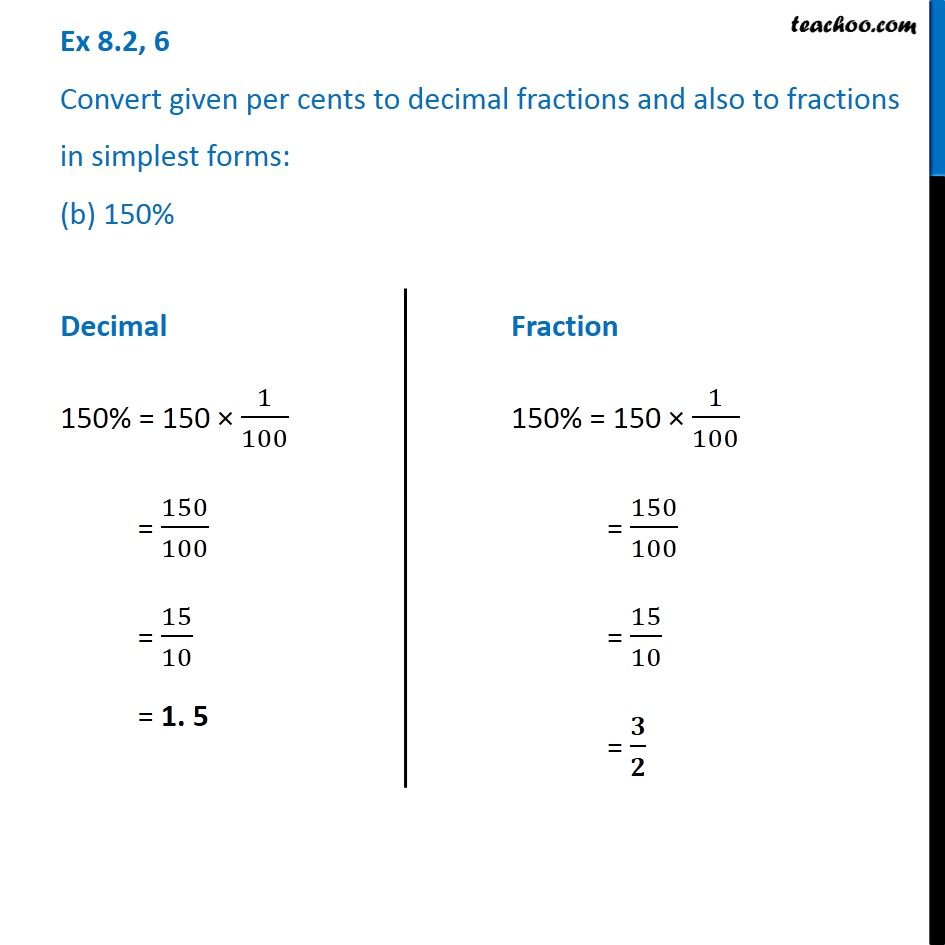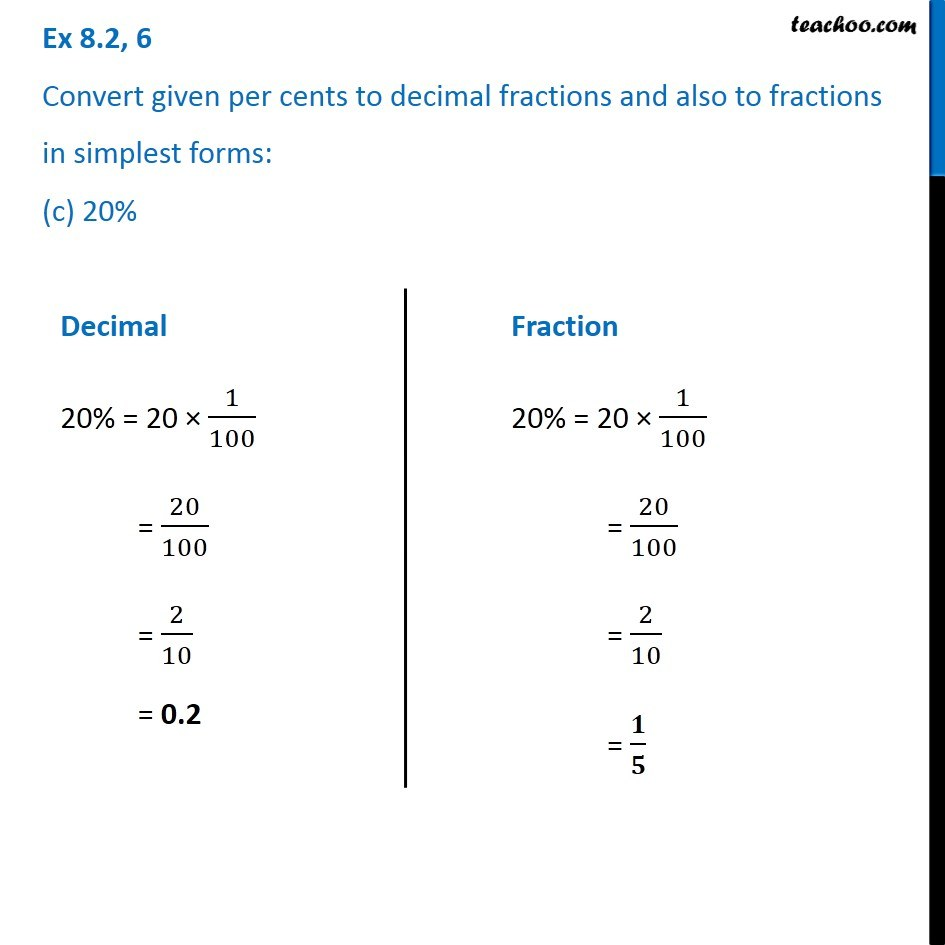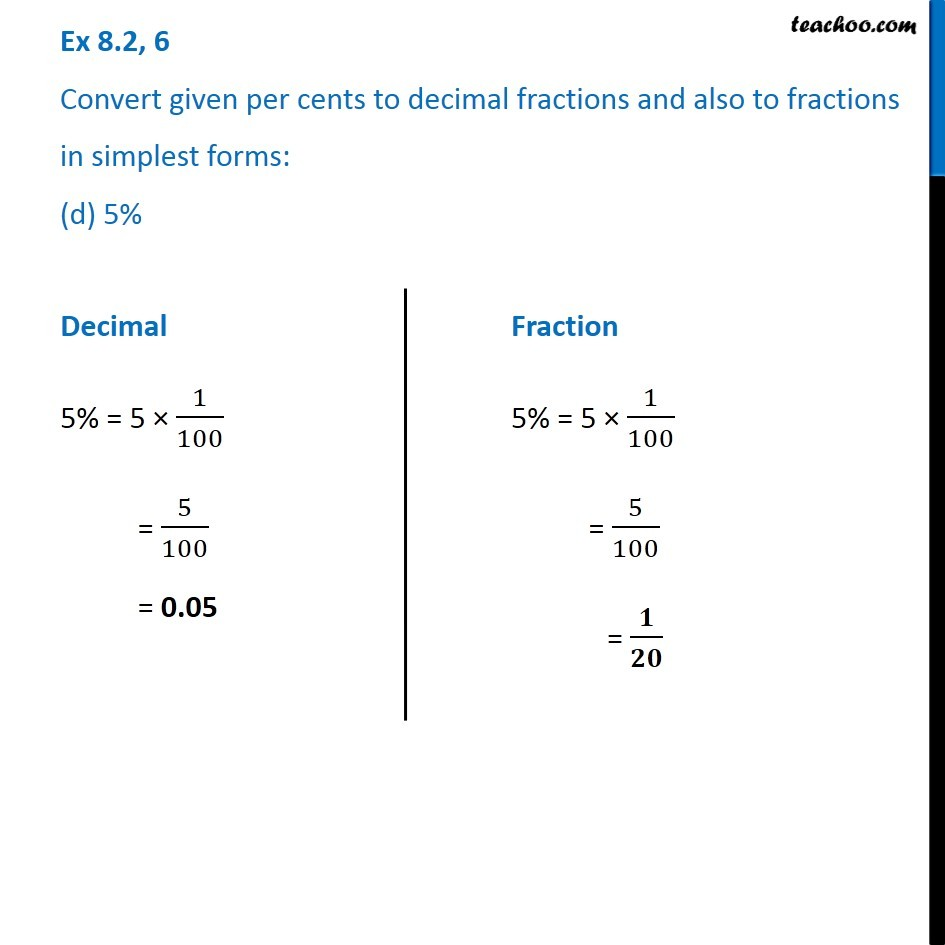1. Chapter 8 Class 7 Comparing Quantities
2. Serial order wise
3. Ex 8.2

Transcript

Ex 8.2, 6 Convert given per cents to decimal fractions and also to fractions in simplest forms: (a) 25% Decimal 25% = 25 × 1/100 = 25/100 = 0.25 Fraction 25% = 25 × 1/100 = 25/100 = 5/20 = 𝟏/𝟒 Ex 8.2, 6 Convert given per cents to decimal fractions and also to fractions in simplest forms: (b) 150% Decimal 150% = 150 × 1/100 = 150/100 = 15/10 = 1. 5 Fraction 150% = 150 × 1/100 = 150/100 = 15/10 = 𝟑/𝟐 Ex 8.2, 6 Convert given per cents to decimal fractions and also to fractions in simplest forms: (c) 20% Decimal 20% = 20 × 1/100 = 20/100 = 2/10 = 0.2 Fraction 20% = 20 × 1/100 = 20/100 = 2/10 = 𝟏/𝟓 Ex 8.2, 6 Convert given per cents to decimal fractions and also to fractions in simplest forms: (d) 5% Decimal 5% = 5 × 1/100 = 5/100 = 0.05 Fraction 5% = 5 × 1/100 = 5/100 = 𝟏/𝟐𝟎

Ex 8.2

About the AuthorDavneet Singh
Davneet Singh is a graduate from Indian Institute of Technology, Kanpur. He has been teaching from the past 10 years. He provides courses for Maths and Science at Teachoo.